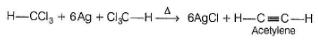Courses

# Test: Dihalides & Polyhalides

## 16 Questions MCQ Test Chemistry Class 12 | Test: Dihalides & Polyhalides

Description
This mock test of Test: Dihalides & Polyhalides for JEE helps you for every JEE entrance exam. This contains 16 Multiple Choice Questions for JEE Test: Dihalides & Polyhalides (mcq) to study with solutions a complete question bank. The solved questions answers in this Test: Dihalides & Polyhalides quiz give you a good mix of easy questions and tough questions. JEE students definitely take this Test: Dihalides & Polyhalides exercise for a better result in the exam. You can find other Test: Dihalides & Polyhalides extra questions, long questions & short questions for JEE on EduRev as well by searching above.
QUESTION: 1

### Only One Option Correct Type Direction (Q. Nos. 1- 13) This section contains 13 multiple choice questions. Each question has four choices (a), (b), (c) and (d), out of which ONLY ONE option is correct. Consider the following reaction,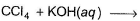Q.  the major product in the above reaction is

Solution: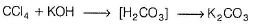QUESTION: 2

### Nitro chloropicrin is prepared-by the action of chloroform and

Solution: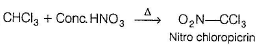QUESTION: 3

### On heating glycerol with excess of HI gives

Solution: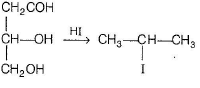QUESTION: 4

A geminal dichloride is formed in all of the following reactions except

Solution: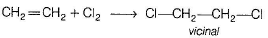QUESTION: 5

A + KOBr → CHBr3 + CH3COOK; Here A is

Solution: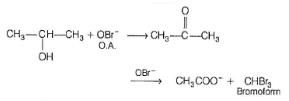QUESTION: 6

The product obtained when bleaching pow der is distilled with acetone

Solution: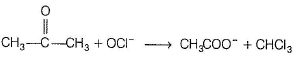QUESTION: 7

What are the products in the following reaction?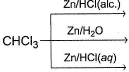Solution:

Reducing power of Zn increases as :
alcohol < H2O < H2O / H+

QUESTION: 8

The end product in the following reaction is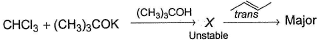Solution: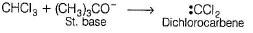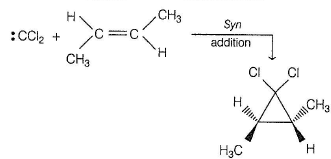QUESTION: 9

What is the product formed in the following reaction?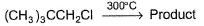Solution:

Alkyl halides on heating undergo rearrangement into higher degree alkyl halide as :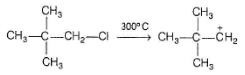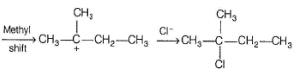QUESTION: 10

What is the major product in the following reaction?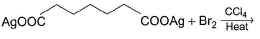Solution:

Hunsdiecker reaction.

QUESTION: 11

What is the major product in the following reaction?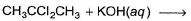Solution: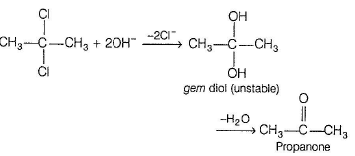QUESTION: 12

What is formed predominantly in the following reaction?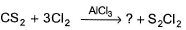Solution:

It is industrial preparation of CCI4.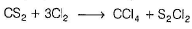QUESTION: 13

The major organic product in the following reaction is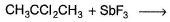Solution:

Swarts reaction-both chlorides are replaced here by fluoride.

*Multiple options can be correct
QUESTION: 14

One or More than One Options Correct Type

Direction (Q. Nos. 14-16) This section contains 3 multiple choice questions. Each question has four choices (a), (b), (c) and (d), out of which ONE or MORE THAN ONE are correct.

Q.

Which upon reaction with Br2in CCI4 gives racemic mixture?

Solution:

Option (a) will give meso dibromide, all other will give racemic dibromide by anti dibromination of double bond.

*Multiple options can be correct
QUESTION: 15

In which of the following reaction(s), reactants and products are correctly matched?

Solution:

Fluorine is not substituted in soivolysis reaction because C—F bond is very strong.

*Multiple options can be correct
QUESTION: 16